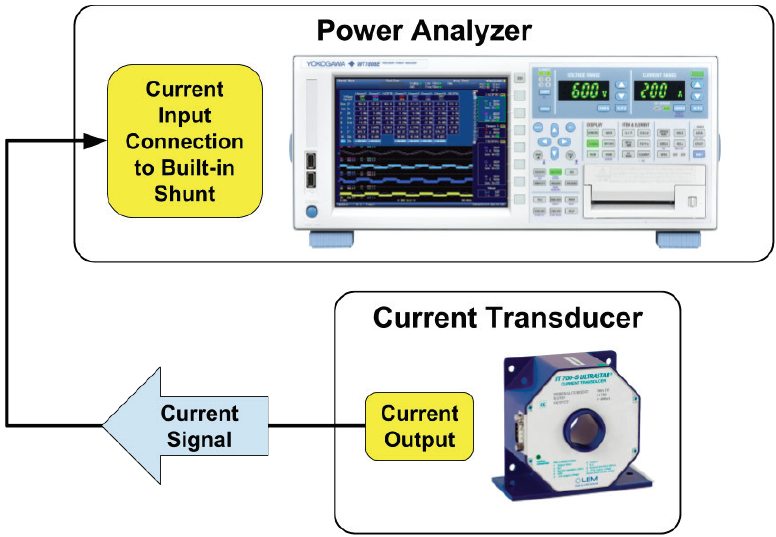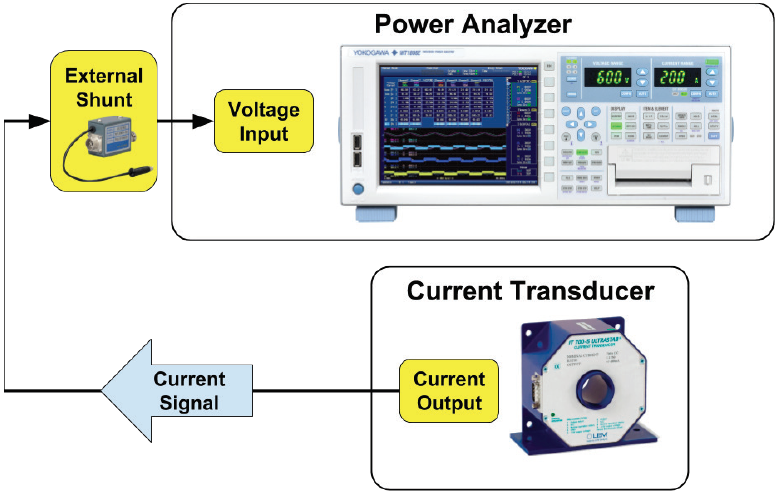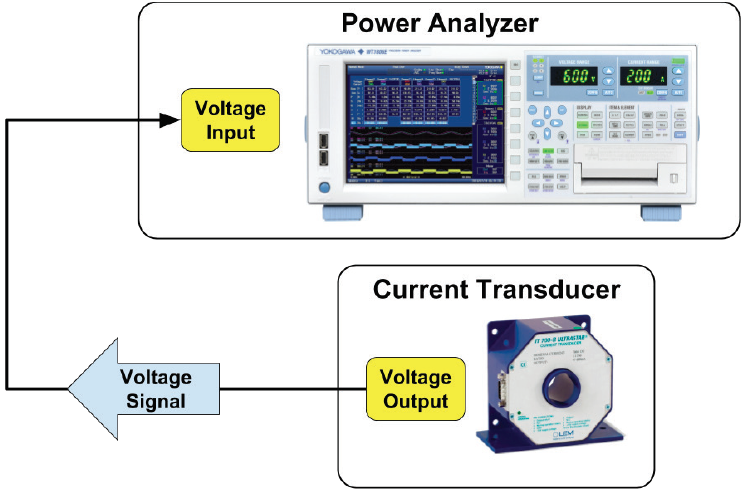# Connecting a Current Transformer to a Power Analyzer

If you are measuring a current that does not exceed the maximum rated input current of the power analyzer you are working with, you can connect the current carrying cable directly to the current inputs of the power analyzer. Many times though, the current to measure will exceed the current input limits of your power analyzer. In these cases you can use a current transformer to convert the current to a voltage or current signal that can be measured directly by the power analyzer.

#### Current Transformer Connection Methods

There are three primary methods of connecting an external Current Transformer / Transducer (CT) to a power analyzer.

Method A - Current output terminals of the CT secondary connect directly to the current input terminals of the power analyzer. The internal shunt on the power analyzer acts as the burden resistor and provides an accurate current measurement that is well characterized. What’s more, transmitting the current from the CT secondary to current input connections has the best noise immunity. Select an input element with a range that maximizes coverage of the range for the current you are measuring. For instance, a 1A maximum input current will use a greater percent of a 2A input element than it would a 30A input element, as the 2A input element supports lower current ranges.Figure 1 - For Method A, the current output of the CT secondary is connected directly to the internal shunt on the power analyzer.

Method B - Current output terminals of the CT secondary connect to an external shunt that is connected directly to a voltage input on the power analyzer. There are cases where this option can supply a superior percent of range.Figure 2 - With method B, a current output of the CT supplies a signal to a burden resistor plugged in to the voltage input of the power analyzer.

Method C - Current output terminals of the CT secondary are terminated directly to a burden resistor close to the CT. The voltage output of the burden resistor is connected to the voltage input of the power analyzer. This method is susceptible to noise and could experience a slight voltage drop depending on the length and location of the cable path.Figure 3 - With method C, a voltage output from the CT supplies a voltage input on the power analyzer directly.

#### the Current-Carrying Cable through the CT to Increase the Precision of your Measurement

With any of these methods, if your current to be measured is less than the available input range of the CT, you can potentially improve the accuracy of your measurement by looping the current carrying cable around the aperture of the CT. Doing so increases the current seen by the CT, thereby using more of the available dynamic range of the CT and improving the measurement accuracy.

Looping the current-carrying cable through the CT aperture multiplies the current seen by the CT by a multiple approximately equal to the number of loops.

Consider an example where you want to measure up to 60A using a 200A CT. If you pass the current-carrying cable once through the CT, you only use 60A of the capacity of the CT, leaving 120A available capacity unused. To make better use of the 200A capacity of your CT, you could loop the 60A cable through the CT three times to achieve a maximum current seen by the CT to 180A, thereby utilizing more of the input range of the CT and increasing the accuracy of your measurement.

### Related Products & Solutions

#### Power Analyzers and Power Meters

Measure characteristics of devices that generate, transform or consume electricity. Also called power meters or wattmeters, these devices measure parameters such as true power (watts), power factor, harmonics, and efficiency.

Top